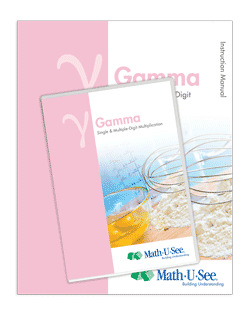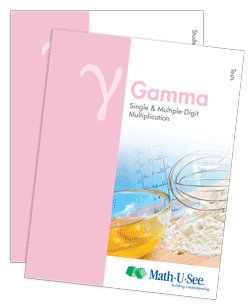# Gamma## Updated Gamma — Available March 2013

Multiplication for Single- and Multiple-Digit Numbers and Other Topics

Once students have mastered the concepts of addition and subtraction (covered in Alpha and Beta), they are ready for multiplication. Gamma teaches single-digit facts and multiple-digit multiplication skills.Updates to the Instruction Manual include introduction to:

• additional ways to express a multiplication problem
• classifying of shapes in more detail
• simple metric measures (no conversions)
• relating solving for the unknown to the idea of division
• adding and subtracting time with no regrouping requiredNew Application & Enrichment pages in the Student Workbook include:

• dot-to-dots featuring all skip counting facts
• using skip counting to make patterns
• pictographs
• logic problems
• Venn diagrams
• recording information on a chart
• arrays
• line graphs
• bar graphs
• line plots
• basic metric measures
• simple fractions
• simple number lines

Major Concepts and Skills Include:

• Using strategies based on place value and properties of operations to multiply
• Fluently multiplying any combination of whole numbers
• Solving for an unknown factor
• Solving abstract and real-world problems involving addition, subtraction, and multiplication
• Measuring and computing area
• Relating concepts of area to addition and multiplication

• Skip counting as a precursor to multiplication
• Adding and subtracting time in hours and minutes
• Multiplying, adding, and subtracting U.S. currency and standard units of measure
• Representing, recording, and interpreting data
• Understanding of basic fractions
• Estimating and solving measurement problems

Check back in the first week of March for ordering information.

Live Chat

9 AM to 6 PM Eastern time,
Monday through Friday

Phone Support

Call (888) 854-6284
International callers —
call +1 717 283 1448

8:30 AM to 6 PM Eastern time,
Monday through Friday

Email